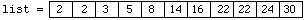This document is part of the HTML publication "An Introduction to the Imperative Part of C++"

The original version was produced by Rob Miller at Imperial College London, September 1996.

Version 1.1 (modified by David Clark at Imperial College London, September 1997)

Version 1.2 (modified by Bob White at Imperial College London, September 1998)

Version 1.3, 1.4, 2.0, ..., 2.21 (modified by William Knottenbelt at Imperial College London, September 1999-September 2022)

# Introduction to C++ Programming: Exercise Sheet 8

## Question 1

The Fibonacci sequence a(1), a(2), a(3), ..., a(n), ... is defined by

• a(1) =1
• a(2) = 1
• a(n) = a(n-1) + a(n-2), for all n > 2

This generates the sequence

1, 1, 2, 3, 5, 8, 13, 21, ...

Write a C++ function "fibonacci(...)" that computes the Fibonacci number corresponding to its positive integer argument, so that, for example, fibonacci(7) == 13.

## Question 2

(a) Write two recursive functions "print_list_forwards(...)" and "print_list_backwards(...)", which print the linked lists from Lecture 7 respectively forwards and backwards on the screen. (The function "print_list_forwards(...)" should behave in exactly the same way as the function "print_list(...)" from Lecture 7). Alter Program 7.5.1 in a suitable way to test the functions. Your program should be able to produce output similar to the following:

```	Enter first word (or '.' to end list): the
Enter next word (or '.' to end list): quick
Enter next word (or '.' to end list): brown
Enter next word (or '.' to end list): fox
Enter next word (or '.' to end list): .

THE LIST FORWARDS IS:
the quick brown fox

THE LIST BACKWARDS IS:
fox brown quick the```

Hint: Look at Program 8.2.1 when designing "print_list_backwards()".

(b) Write and test an iterative (i.e. non-recursive) version of the function "print_list_backwards(...)". (Which definition did you find easier?)

## Question 3

Given positive two integers m and n such that m<n, the greatest common divisor of m and n is the same as the greatest common divisor of m and (n-m). Use this fact to write a recursive definition of the function "greatest_common_divisor(...)", which takes two positive integer arguments and returns their greatest common divisor. Test your function in a suitable main program.

## Question 4

Binary Search. Binary Search is recursively defined algorithm for searching through a sorted list (array) to find a position of a particular value. It is particularly efficient for large lists, since it avoids the need to search sequentially (i.e. one value at a time).

The idea is as follows. Suppose we want to find a position of the value v in the previously sorted array a[]. We first look at the value stored half way along a[]. If this happens to be v, we are finished, and we can simply return this middle position. However, if the value at the middle position is less than v, we now know we only have to search the 2nd half of the list, and so we repeat the search procedure just with this half of the list. Similarly, if the value at the middle position is greater than v, we now know we only have to search the 1st half of the list. (Imagine looking for the page containing the definition for a particular word in a dictionary. If using binary search, you would start at the middle page, and then just restrict your attention either to the part of the dictionary before this page, or the half after this page, depending on what words you saw on the middle page.) Binary search is discussed in more detail in Savitch, Section 14.3.

Write a function with function declaration

`	int binary_search(int value, int list[], int first, int last);`

which searches the values in the array "list[]" from "list[first]" to "list[last]" to find an array element with value "value". If it finds "value", it returns the position of "value" in "list[]". Otherwise it returns -1. For example, given the arraythe call

`	binary_search(24, list, 0, 10);`

returns the value 9, the call

`	binary_search(24, list, 2, 6);`

returns the value -1, and the call

`	binary_search(22, list, 0, 10);`

either returns the value 7 or returns the value 8.

Test your function in a suitable main program. (You could simply extend Program 8.7.1).